Question

# Three very long, straight parallel wires each carry currents of I, directed out of the page....

Three very long, straight parallel wires each carry currents of I, directed out of the page. The wires pass through the vertices of a right isosceles triangle of side d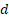. What is the magnitude of the magnetic field at a point P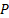at the midpoint of the hypotenuse of the triangle?

There is no diagram.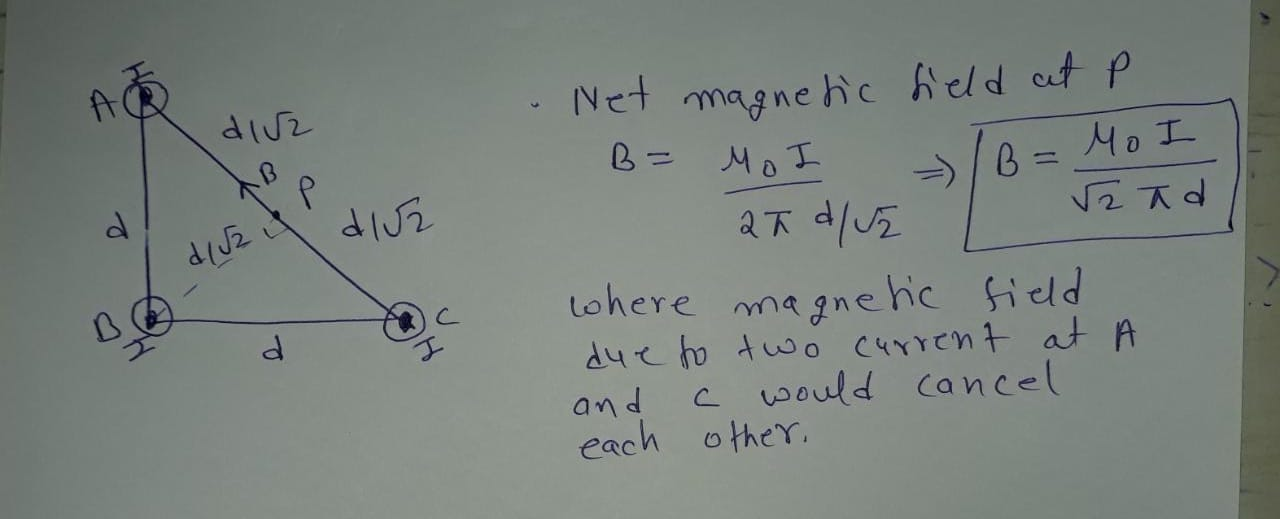#### Earn Coins

Coins can be redeemed for fabulous gifts.

Similar Homework Help Questions
• ### Please explain all steps, formulas, and concepts involved. currents of 4.00 A, directed out of the...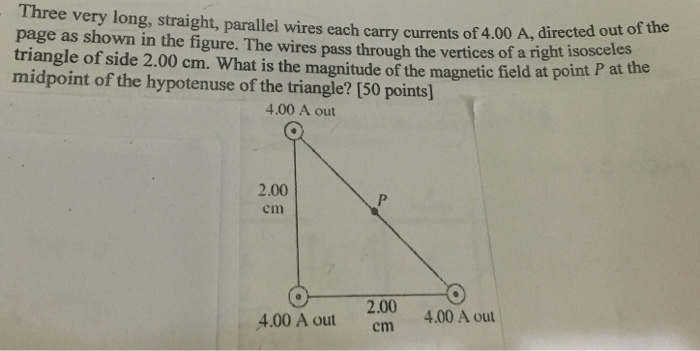Please explain all steps, formulas, and concepts involved. currents of 4.00 A, directed out of the Three very long, straight, parallel wires each carry currents of 4.00 A, directe page as shown in the figure. The wires pass through the vertices of a nght triangle of side 2.00 cm. What is the magnitude of the magnetic field at point midpoint of the hypotenuse of the triangle? (50 points) 4.00 A out O 2.00 cm 2.00 4.00 A outcm 4.00 A...

• ### Two very long straight parallel wires each carry currents as shown in the figure a point...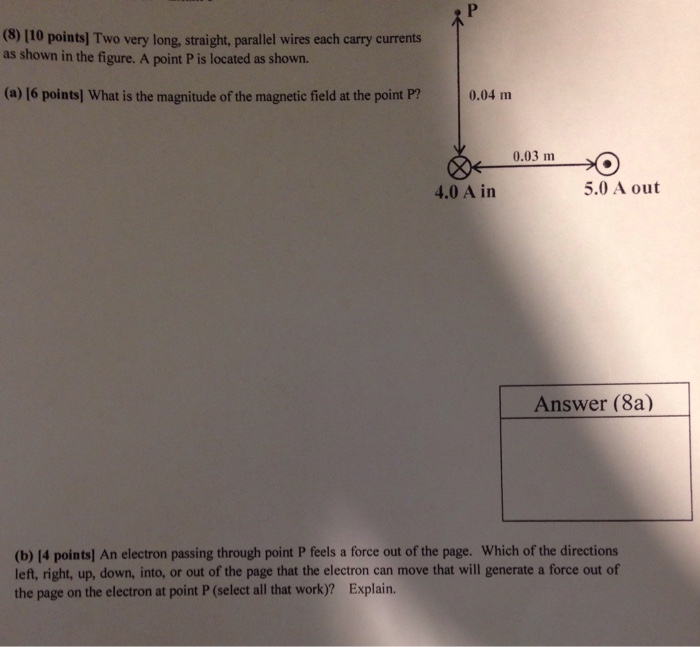Two very long straight parallel wires each carry currents as shown in the figure a point P is located as shown. A) what is the magnitude of the magnetic field at point P? B) Ab electron passing through point P feels a forced out of the page. Which of the directions left, right, up, down, into, or out of the page that the electron can move that will generate a force out of the page on the electron at point...

• ### Two very long, straight, parallel wires carry currents that are directed perpendicular to the page as...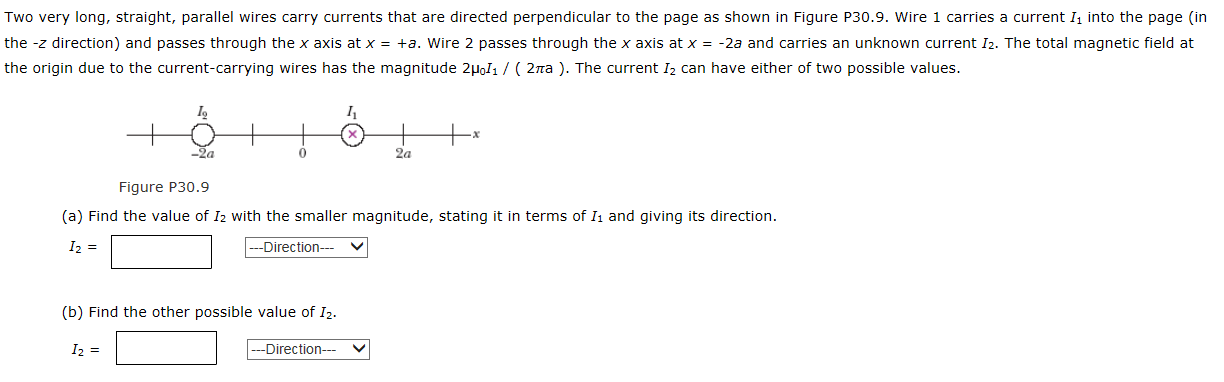Two very long, straight, parallel wires carry currents that are directed perpendicular to the page as shown in Figure P30.9. Wire 1 carries a current 1. into the page (in the -z direction) and passes through the x axis at x = -2a. Wire 2 passes through the x axis at x = -+a and carries an unknown current 12. The total magnetic field at the origin due to the current-carrying wires has the magnitude 2PoIi / ( 2 pira...

• ### Three long straight wires are parallel to each other and carry currents directed into or out...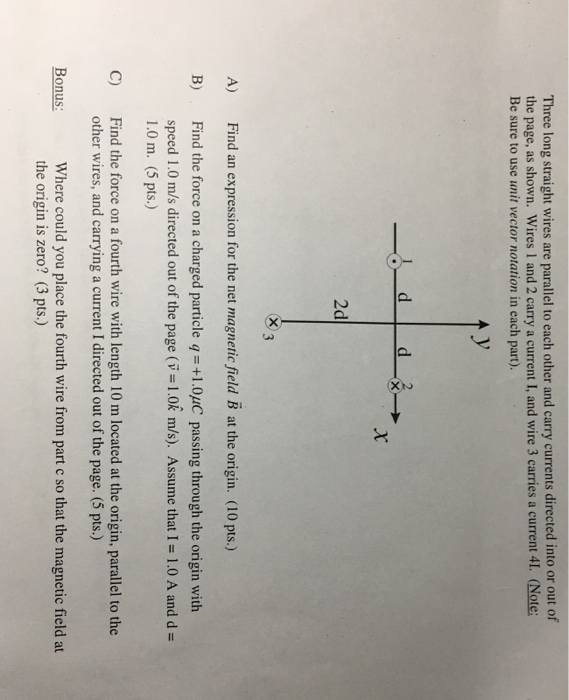Three long straight wires are parallel to each other and carry currents directed into or out of the page, as shown. Wires 1 and 2 carry a current I, and wire 3 carries a current 41. (Note: Be sure to use unit vector notation in each part). 2d indn rssio for th net magnaie fald B ) B) Find the force on a charged particle q-+1.0uC passing through the origin with speed 1.0 m/s directed out of the page (v-1.0k...

• ### Problem 1 Three long straight wires are parallel to each other and carry currents directed into...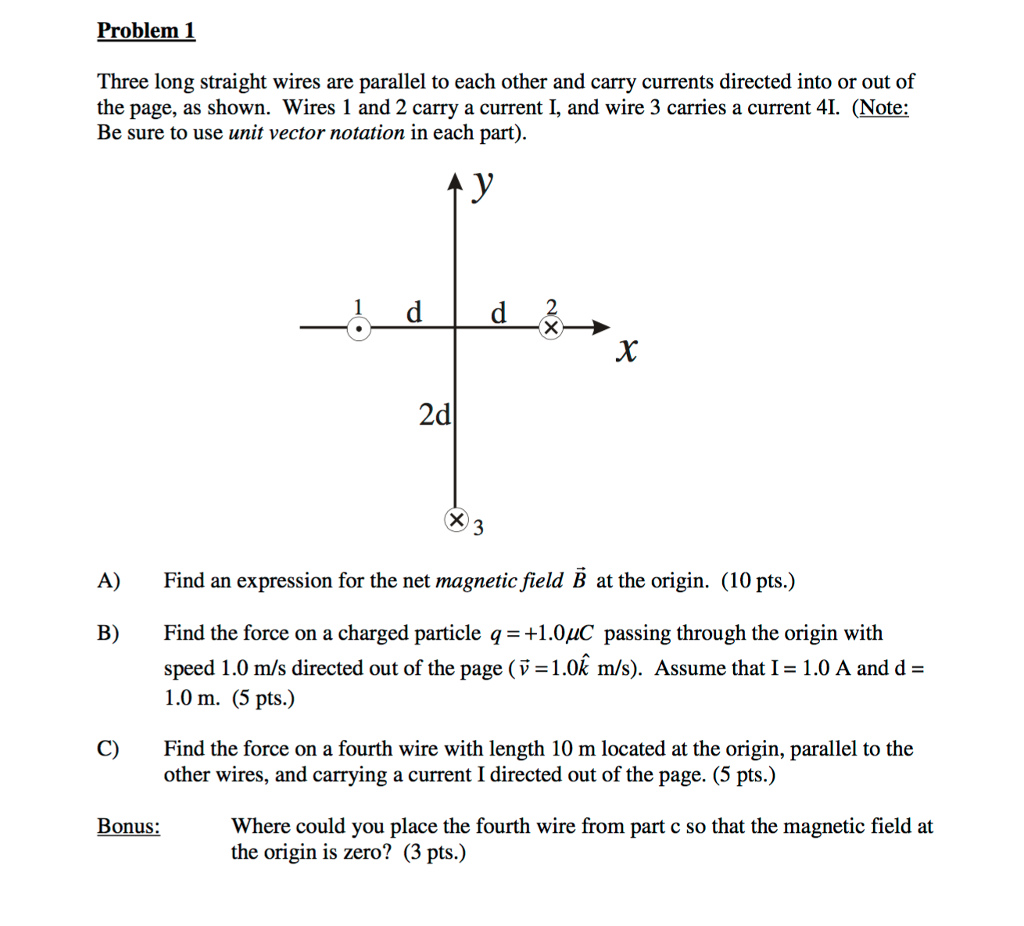Problem 1 Three long straight wires are parallel to each other and carry currents directed into or out of the page, as shown. Wires 1 and 2 carry a current I, and wire 3 carries a current 4. (Note: Be sure to use unit vector notation in each part) 2 2d A) Find an expression for the net magnetic field B at the origin. (10 pts.) B) Find the force on a charged particle q1.0uC passing through the origin with...

• ### 5. (5 points) Two very long, straight parallel wires carry currents of equal magnitude, but opposite...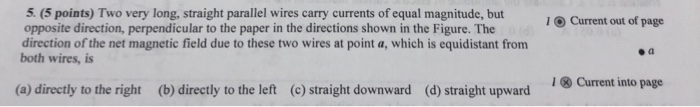5. (5 points) Two very long, straight parallel wires carry currents of equal magnitude, but opposite direction, perpendicular to the paper in the directions shown in the Figure. The direction of the net magnetic field due to these two wires at point a, which is equidistant from both wires, is 1O Current out of page I ⓧ Current into page (a) directly to the right (b) directly to the left (c) straight downward (d) straight upward

• ### Two very long straight parallel wires carry currents 12 = 25 A and I2 = 30...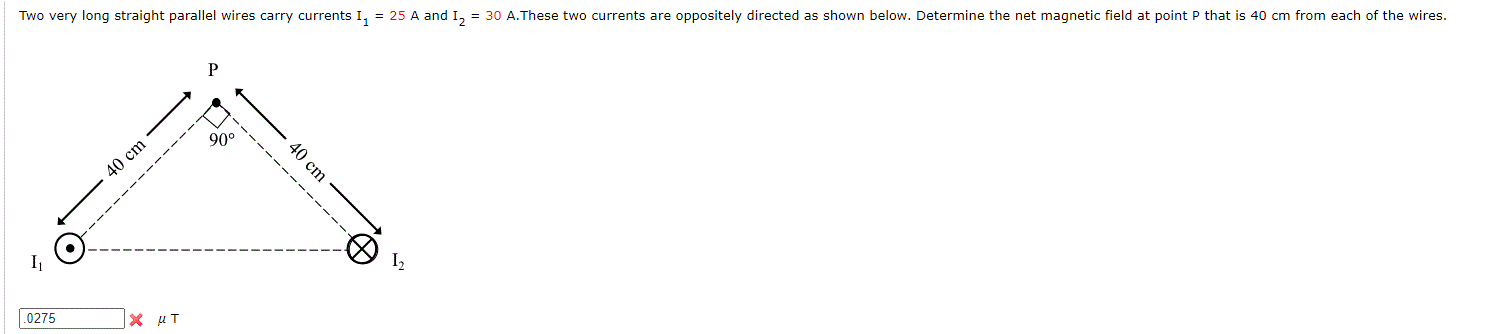Two very long straight parallel wires carry currents 12 = 25 A and I2 = 30 A.These two currents are oppositely directed as shown below. Determine the net magnetic field at point P that is 40 cm from each of the wires. Р 90° 40 cm 40 cm ------ ------------- 1 12 .0275 X UT

• ### Two very long, straight, parallel wires separated by distance d carry currents of magnitude I in...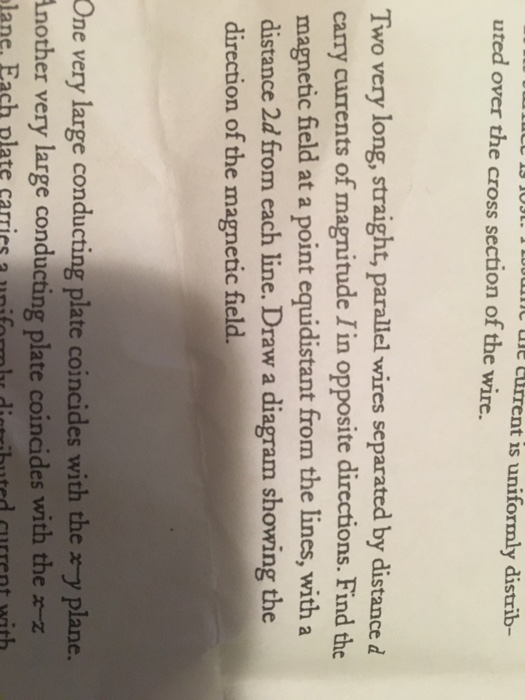Two very long, straight, parallel wires separated by distance d carry currents of magnitude I in opposite directions. Find the magnetic field at a point equidistant from the lines, with a distance 2d from each line. Draw a diagram showing the direction of the magnetic field. One very large conducting plate coincides with the x-y plane.

• ### The figure below shows two long, parallel wires incross-section, and the currents they carry are...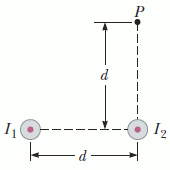The figure below shows two long, parallel wires in cross-section, and the currents they carry are directed out of the page. The left wire carries a current ofI1 = 2.96 A,and the right carries a current ofI2 = 3.45 A.The two wires are a distanced = 20.0 cmapart. Point P is the same distance d above the right wire.Assume the +x-direction is to the right, and the +y-direction is upward.A cross-sectional end view of two parallel wires carrying current out...

• ### Two very long straight parallel wires carry currentsI1 = 30 A and I2 = 35...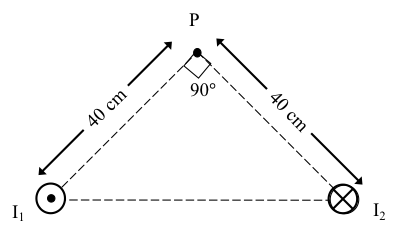Two very long straight parallel wires carry currents I1 = 30 A and I2 = 35 A.These two currents are oppositely directed as shown below. Determine the net magnetic field at point P that is 40 cm from each of the wires.